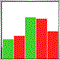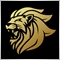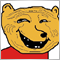# Stop / kill calculating a function.540

Hello again,
I need to stop calculating a function because of CPU.

I created a BUTTON.
TRUE state => I call + dysplay my_function.
FALSE state => I hide the dysplayed grapical objects and indications. (but the function is still there and take CPU ressources).

How can I stop / kill the function's calculation ?

Regards.540

Really no way to kill a function ?Moderator
12383

Finish the calculation (cycle) and then decide whether to process the result further or not.

There are trillions of true/false a second, this is how a computer operates.1077

Where is that function, in OnCalculate or OnEvent?540

kypa:
Where is that function, in OnCalculate or OnEvent?

Hello,
In "OnCalculate".540

Here is the illustration of what i want to do :
```void Function_Already_in_OnCalculate()
{
bool My_Button_State=ObjectGetInteger(0,"My_Button",OBJPROP_STATE);

if(My_Button_State==true)
{
My_Called_Function();
}

if(My_Button_State==false)
{
// Kill My_Called_Function
}
}```
How can i do that the simpler way please ?Moderator
12383

You mean slower ?

Then you can put it in a timer so you can define the interval in MilliSeconds.540

Marco vd Heijden:

You mean slower ?

Then you can put it in a timer so you can define the interval in MilliSeconds.

Hello,
I mean really kill it.
Like it's never existed, and no calculated anymore.Moderator
12383

It's the correct approach true or false.

But you can not go back in time to undo the calculation if it already started if that is what you mean.

Probably just need optimization to make it more efficient.

Maybe you can enlighten us as to why you want to 'kill' the function ?540

I dysplay highs and lows of many timeframes with parameters "Processed_Periods".
But even if delete all objects, the calculation is still runing.

Maybe the best way,is to change "Processed_Periods = 0;" when button is FALSE. (?)540

Like this :
```if(TLR_Button_Periods_Ranges_State==false)
{
Periods_Ranges_To_Process=0;
ObjectDeleteAll();
}```
...
It calls a array. How can I nullify an Array ?
```double m_MN1[];
ArrayCopyRates(m_MN1,Symbol(),PERIOD_MN1);```